SLVSET3D March   2020  – October 2020

PRODUCTION DATA

1. Features
2. Applications
3. Description
4. Revision History
5. Pin Configuration and Functions
6. Specifications
7. Detailed Description
1. 7.1 Overview
2. 7.2 Functional Block Diagram
3. 7.3 Feature Description
4. 7.4 Device Functional Modes
8. Application and Implementation
1. 8.1 Application Information
2. 8.2 Typical Application
1. 8.2.1 Design Requirements
2. 8.2.2 Detailed Design Procedure
3. 8.2.3 Application Curves
9. Power Supply Recommendations
10. 10Layout
11. 11Device and Documentation Support
12. 12Mechanical, Packaging, and Orderable Information

• DSK|10
• DSK|10

### 8.2.3 Application Curves

Table 8-5 Components for Application Characteristic Curves for VOUT = 3.3 V
REFERENCE(1) DESCRIPTION(2) PART NUMBER MANUFACTURER
U1 400-mA ultra low Iq Buck-Boost Converter (2.5 mm x 2.5 mm QFN) TPS63900DSK Texas Instruments
L1 2.2 µH, 2.5 mm x 2 mm x 1.2 mm, 3.3 A, 82 mΩ DFE252012F-2R2M Murata
C1 10 µF, 0603, Ceramic Capacitor, ±20%, 6.3 V GRM188R60J106ME47 Murata
C2 22 µF, 0603, Ceramic Capacitor, ±20%, 6.3 V GRM187R60J226ME15 Murata
CFG1 36.5 kΩ, 0603 Resistor, 1%, 100 mW Standard Standard
CFG2 0 Ω, 0603 Resistor, 1%, 100 mW Standard Standard
CFG3 0 Ω, 0603 Resistor, 1%, 100 mW Standard Standard
For other output voltages, refer to Table 8-1 for resistor values.
Table 8-6 Typical Characteristics Curves
PARAMETER CONDITIONS FIGURE
Output Current Capability
Typical Output Current Capability versus Input Voltage VO = 1.8 V to 5.0 V Figure 8-2
Switching Frequency
Typical Burst Switching Frequency versus Output Current VI = 3.3 V, VO = 1.8 V to 5.0 V Figure 8-3
Typical Burst Switching Frequency versus Output Current VI = 2.0 V, VO = 1.8 V to 5.0 V Figure 8-4
Typical Burst Switching Frequency versus Output Current VI = 5.2 V, VO = 1.8 V to 5.0 V Figure 8-5
Efficiency
Efficiency versus Output Current VI = 1.8 V to 5.5 V, VO = 1.8 V Figure 8-6
Efficiency versus Output Current VI = 1.8 V to 5.5 V, VO = 3.3 V Figure 8-7
Efficiency versus Output Current VI = 1.8 V to 5.5 V, VO = 5.0 V Figure 8-8
Efficiency versus Input Voltage IO = 1 μA to 400 mA, VO = 3.3 V Figure 8-9
Switching Waveforms
Switching Waveforms, Boost Operation VI = 1.8 V, VO = 3.3 V Figure 8-10
Switching Waveforms, Boost Operation VI = 2.8 V, VO = 3.3 V Figure 8-11
Switching Waveforms, Buck-Boost Operation VI = 3.3 V, VO = 3.3 V Figure 8-12
Switching Waveforms, Buck Operation VI = 4.0 V, VO = 3.3 V Figure 8-13
Output Voltage Ripple
Output Voltage Ripple VI = 2.0 V, VO = 1.8 V to 5.0 V Figure 8-14
Output Voltage Ripple VI = 3.3 V, VO = 1.8 V to 5.0 V Figure 8-15
Output Voltage Ripple VI = 5.2 V, VO = 1.8 V to 5.0 V Figure 8-16
Output Voltage Ripple over Temperature VI = 3.3 V, VO = 3.6 V Figure 8-17
Regulation Accuracy
Load Regulation VO = 3.3 V Figure 8-18
Line Regulation VI = 1.8 V to 5.0 V, Load = 1 mA Figure 8-19
Transient Performance
Line Transient, Light Load VI = 2.5 V to 4.2 V, VO = 3.3 V, Load = 1 mA Figure 8-20
Line Transient, High Load VI = 2.5 V to 4.2 V, VO = 3.3 V, Load = 100 mA Figure 8-21
Load Transient, 100 mA Step VI = 1.8 V, VO = 3.3 V, Load = 0 mA to 100 mA Figure 8-22
Load Transient, 100 mA Step VI = 3.3 V, VO = 3.3 V, Load = 0 mA to 100 mA Figure 8-23
Load Transient, 100 mA Step VI = 1.8 V, VO = 3.3 V, Load = 0 mA to 100 mA Figure 8-24
Load Transient, 300 mA Step VI = 3.3 V, VO = 1.8 V, Load = 0 mA to 300 mA Figure 8-25
Load Transient, 300 mA Step VI = 3.3 V, VO = 3.3 V, Load = 0 mA to 300 mA Figure 8-26
Load Transient, 300 mA Step VI = 5.5 V, VO = 3.3 V, Load = 0 mA to 300 mA Figure 8-27
Start-up
Start-up Behavior from Rising Enable VI = 3.3 V, VO = 3.3 V, Load = 100 mA Figure 8-28
Start-up Behavior from Rising Enable VI = 1.8 V, VO = 1.8 V, Load = 10 μA Figure 8-29
Start-up Behavior from Rising Enable VI = 1.8 V, VO = 5.0 V, Load = 10 μA Figure 8-30
Start-up Behavior from Rising Enable VI = 1.8 V, VO = 5.0 V, Load = 1000 μF Figure 8-31
ICL (Input Current Limit)
Start-up with 1 mA ICL VI = 3.3 V, VO = 3.3 V, CO = 300 μF Figure 8-32
Start-up with 2.5 mA ICL VI = 3.3 V, VO = 3.3 V, CO = 300 μF Figure 8-33
Start-up with 5 mA ICL VI = 3.3 V, VO = 3.3 V, CO = 300 μF Figure 8-34
Start-up with 10 mA ICL VI = 3.3 V, VO = 3.3 V, CO = 300 μF Figure 8-35
Start-up with 25 mA ICL VI = 3.3 V, VO = 3.3 V, CO = 300 μF Figure 8-36
Start-up with 50 mA ICL VI = 3.3 V, VO = 3.3 V, CO = 300 μF Figure 8-37
Start-up with 100 mA ICL VI = 3.3 V, VO = 3.3 V, CO = 300 μF Figure 8-38
Short Circuit Behavior
Short Circuit Behavior VI = 3.3 V, VO = 1.8 V Figure 8-39
Short Circuit Behavior VI = 3.3 V, VO = 3.3 V Figure 8-40
Short Circuit Behavior VI = 3.3 V, VO = 5.0 V Figure 8-41
DVS (Digital Voltage Scaling)
DVS Behavior at Light Load VI = 3.3 V, VO(1) = 2.2 V, VO(2) = 3.6 V, Load = 1 kΩ Figure 8-42
DVS Behavior at High Load VI = 3.3 V, VO(1) = 2.2 V, VO(2) = 3.6 V, Load = 30 Ω Figure 8-43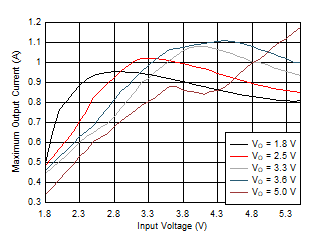TA = 25°C
Figure 8-2 Typical Output Current Capability versus Input Voltage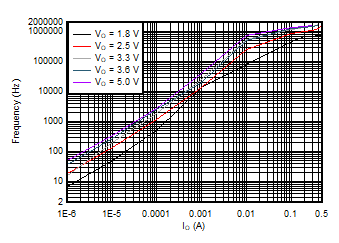VI = 2.0 V TA = 25°C
Figure 8-4 Typical Burst Switching Frequency versus Output Current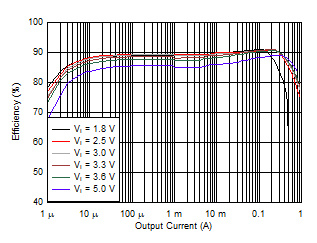VO = 1.8 V TA = 25°C
Figure 8-6 Efficiency versus Output Current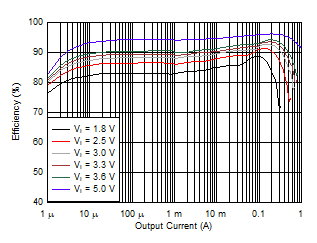VO = 5.0 V TA = 25°C
Figure 8-8 Efficiency versus Output Current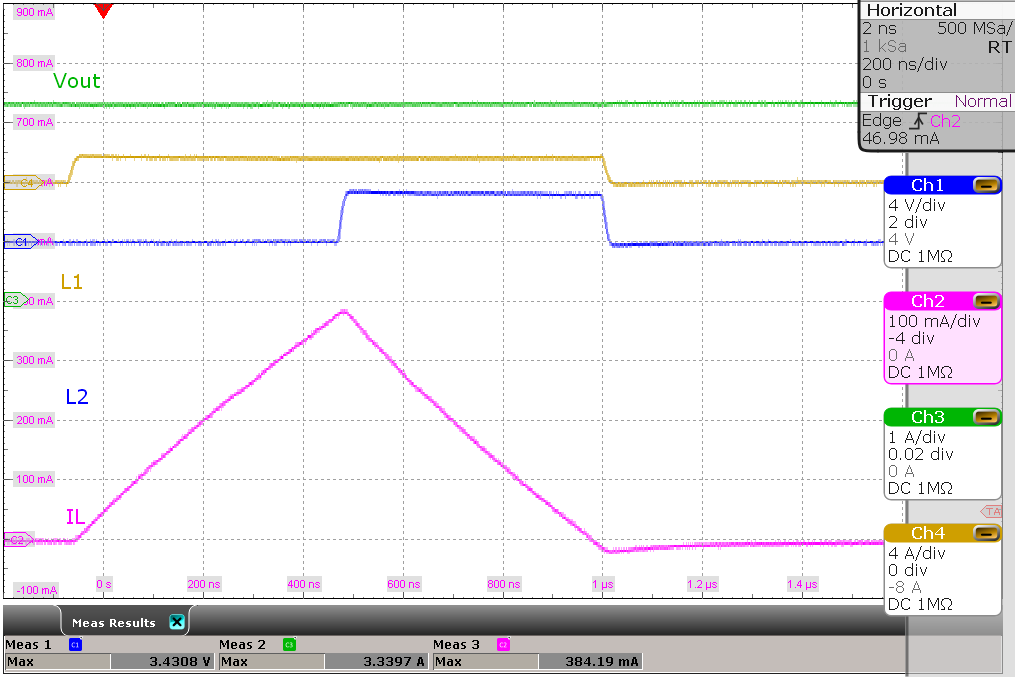VI = 1.8 V, VO = 3.3 V No load
Figure 8-10 Switching Waveforms, Boost Operation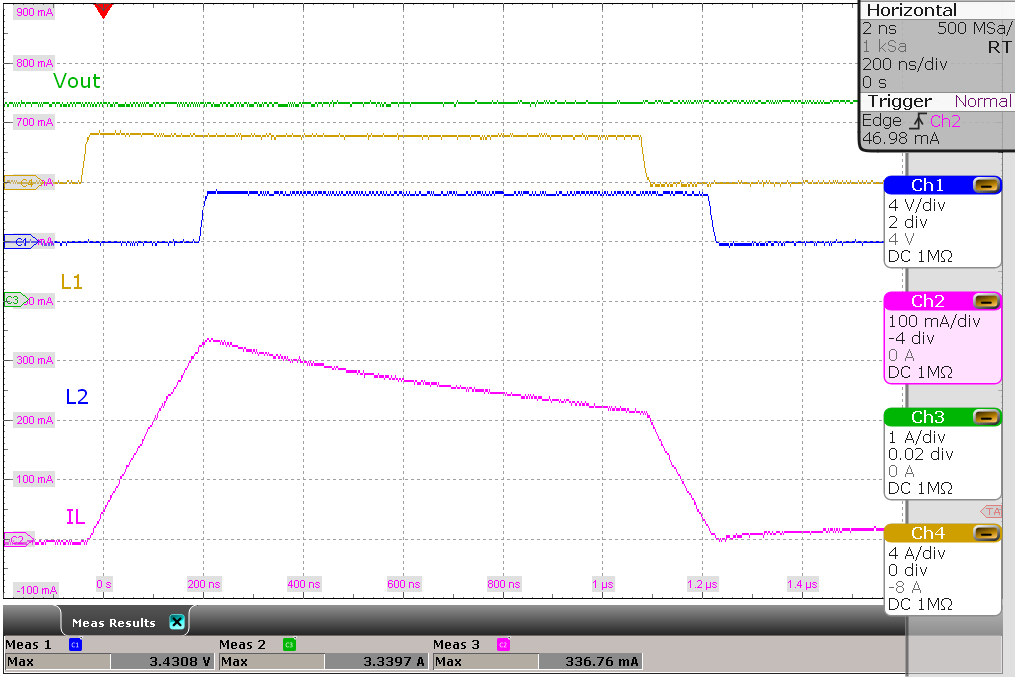VI = 3.3 V, VO = 3.3 V No load
Figure 8-12 Switching Waveforms, Buck-Boost Operation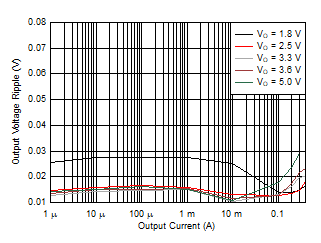VI = 2.0 V TA = 25°C
Figure 8-14 Output Voltage Ripple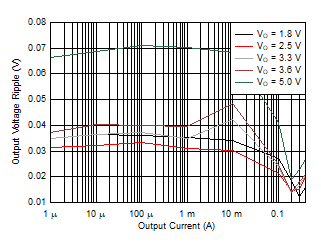VI = 5.2 V TA = 25°C
Figure 8-16 Output Voltage Ripple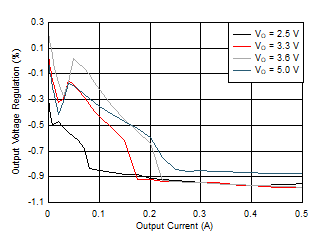VO = 3.3 V TA = 25°C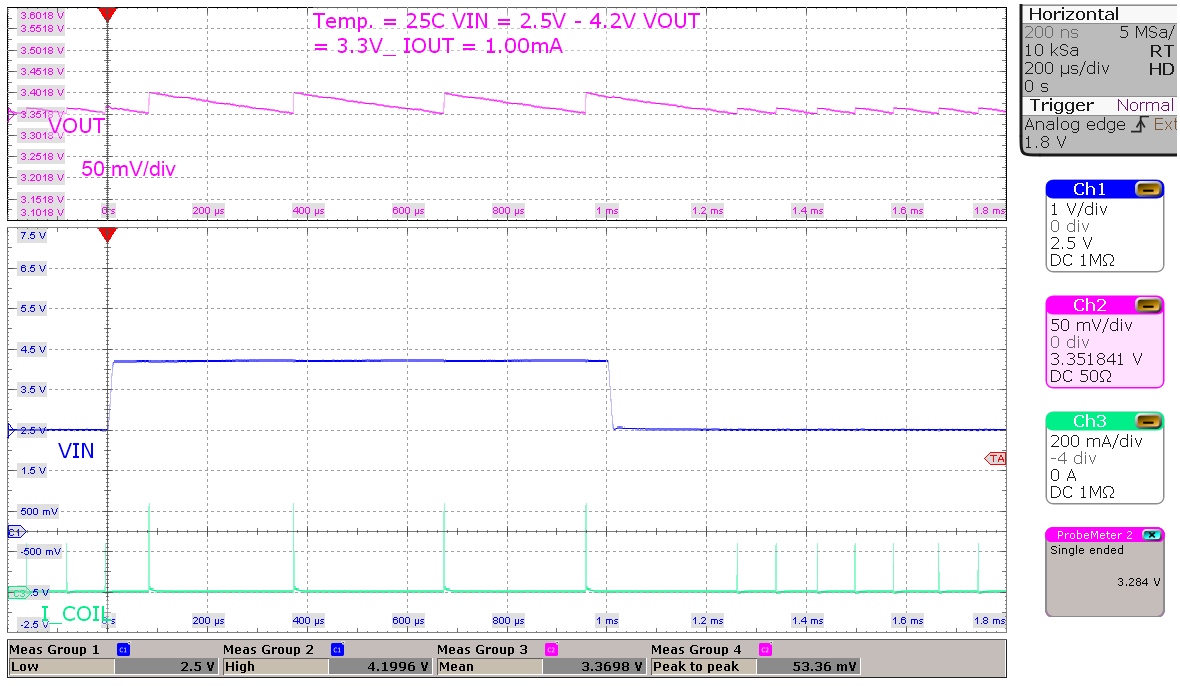VI = 2.5 V to 4.2 V, VO = 3.3V Load = 1 mA
Figure 8-20 Line Transient, Light Load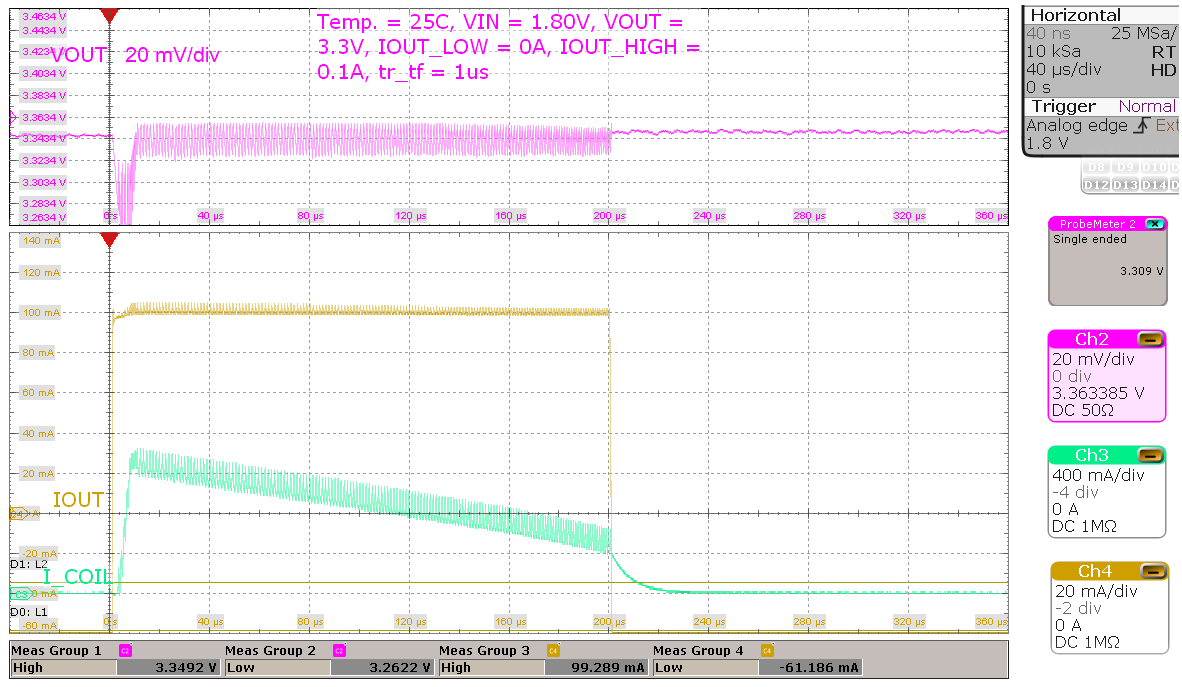VI = 1.8 V, VO = 3.3 V Load = 0 mA to 100 mA, tr/tf = 1 μs
Figure 8-22 Load Transient, 100 mA Step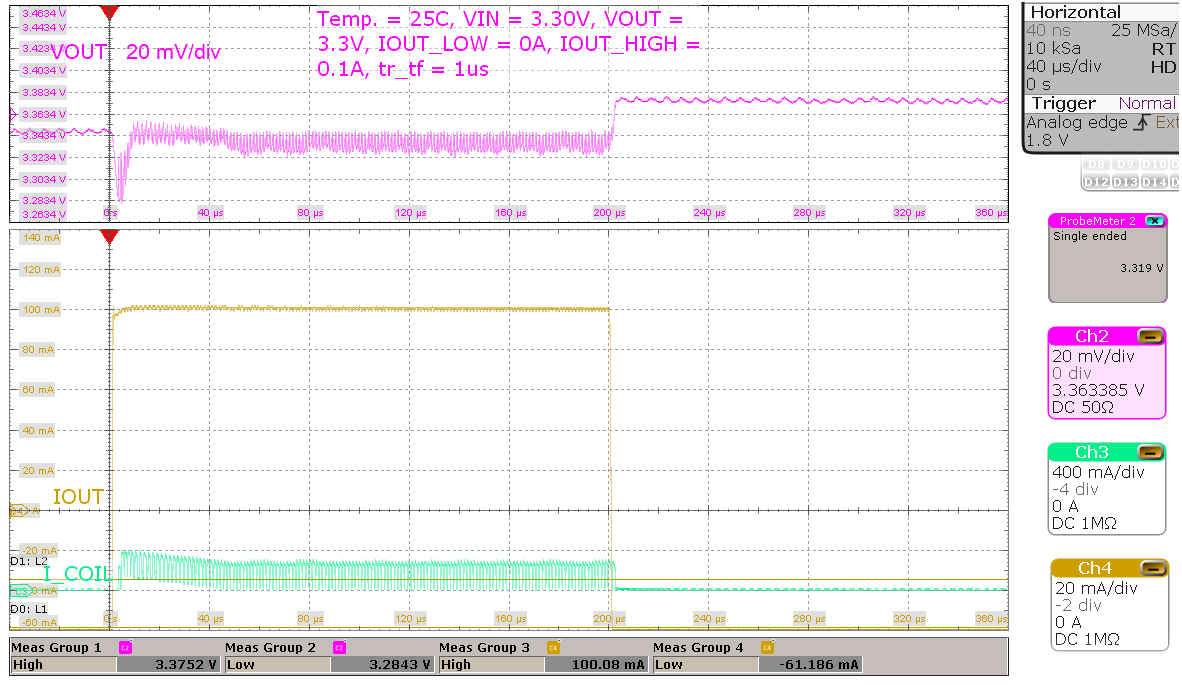VI = 3.3 V, VO = 3.3 V Load = 0 mA to 100 mA, tr/tf = 1 μs
Figure 8-24 Load Transient, 100 mA Step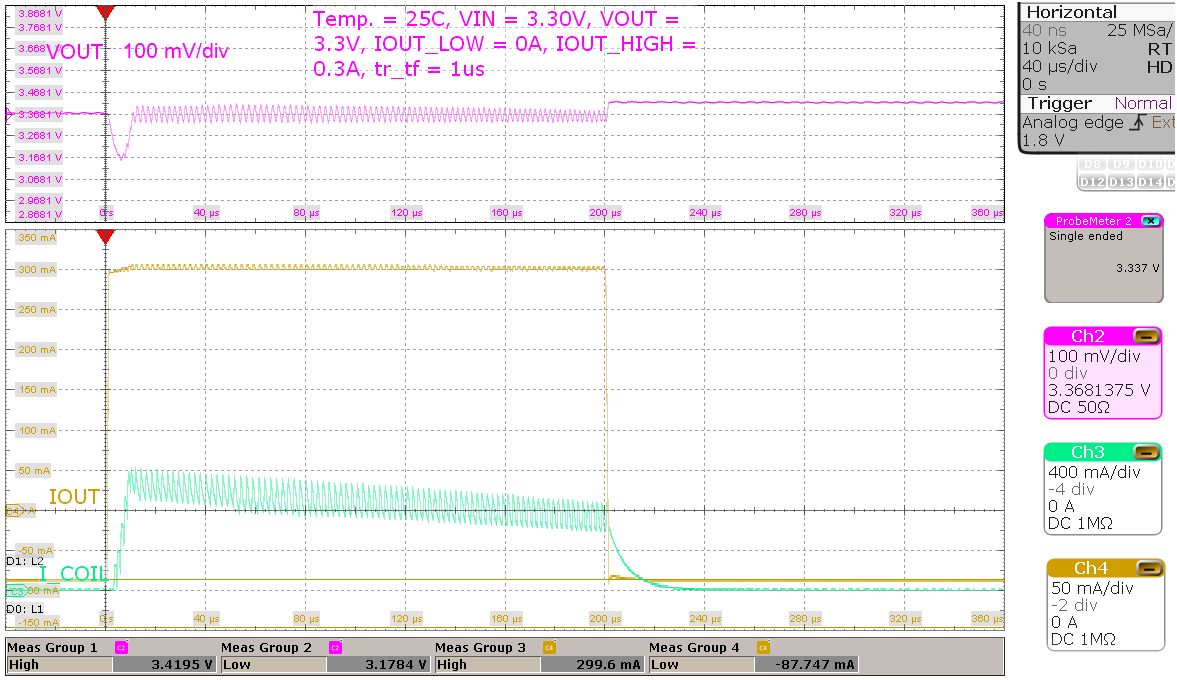VI = 3.3 V, VO = 3.3 V Load = 0 mA to 300 mA, tr/tf = 1 μs
Figure 8-26 Load Transient, 300 mA Step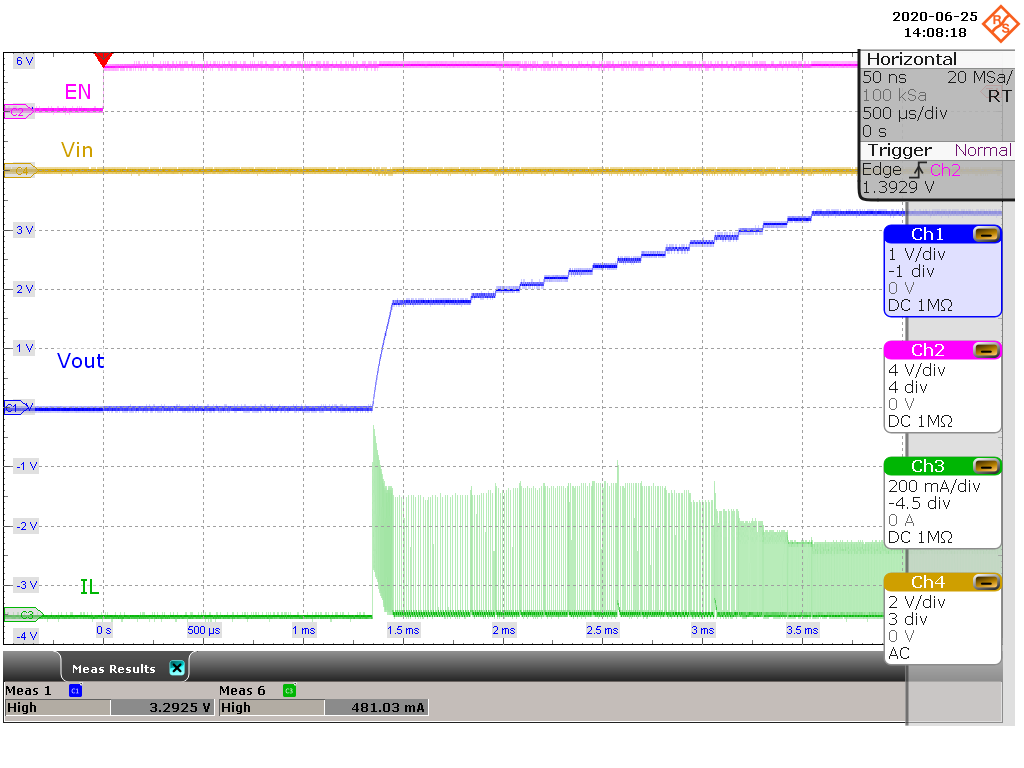VI = 3.3 V, VO = 3.3 V 100-mA resistive load
Figure 8-28 Start-up Behavior from Rising Enable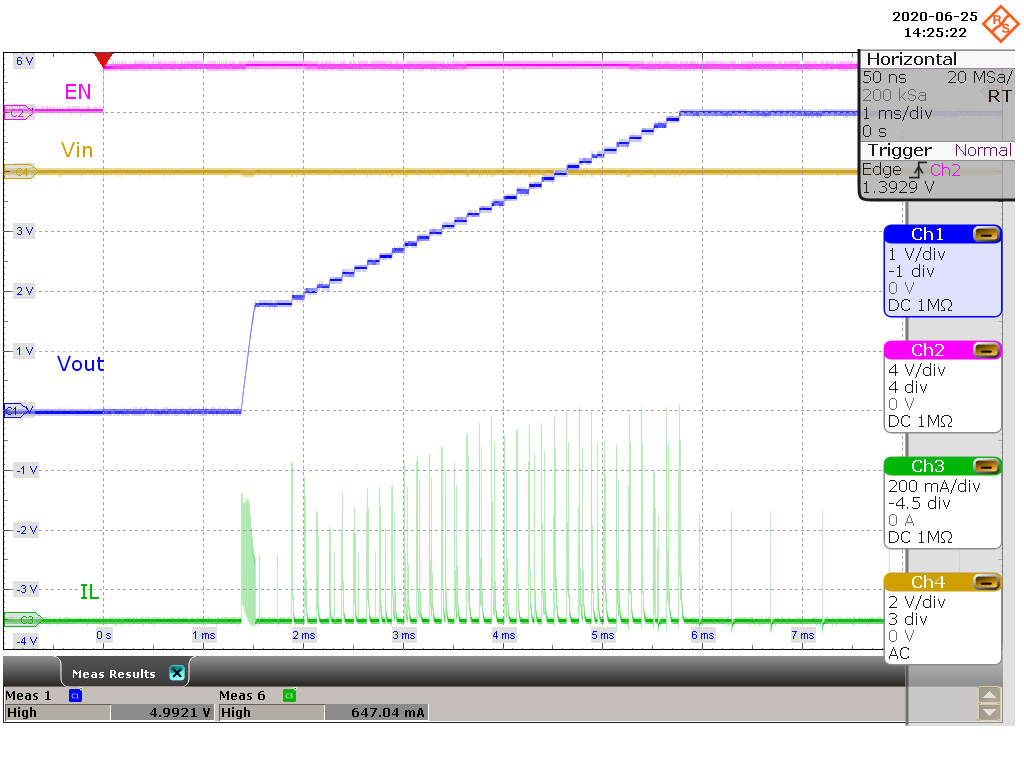VI = 1.8 V, VO = 5.0 V 10-μA resistive load
Figure 8-30 Start-up Behavior from Rising Enable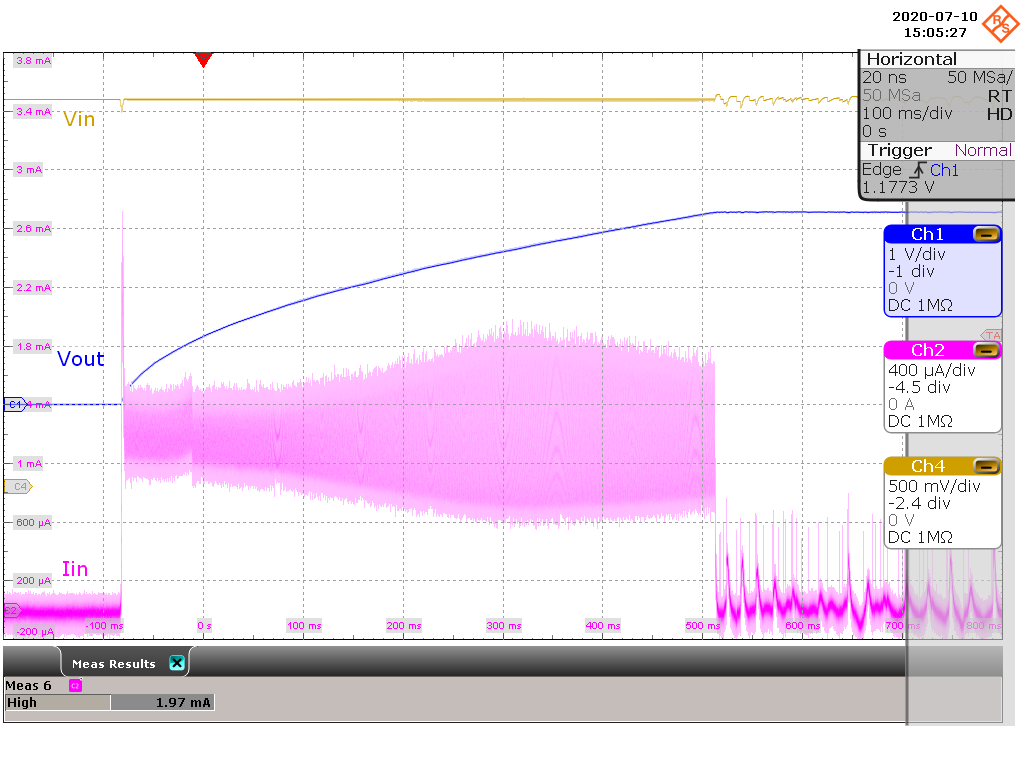VI = 3.3 V, VO = 3.3 V CI = 32 μF, CO = 300 μF
Figure 8-32 Start-up with 1-mA ICL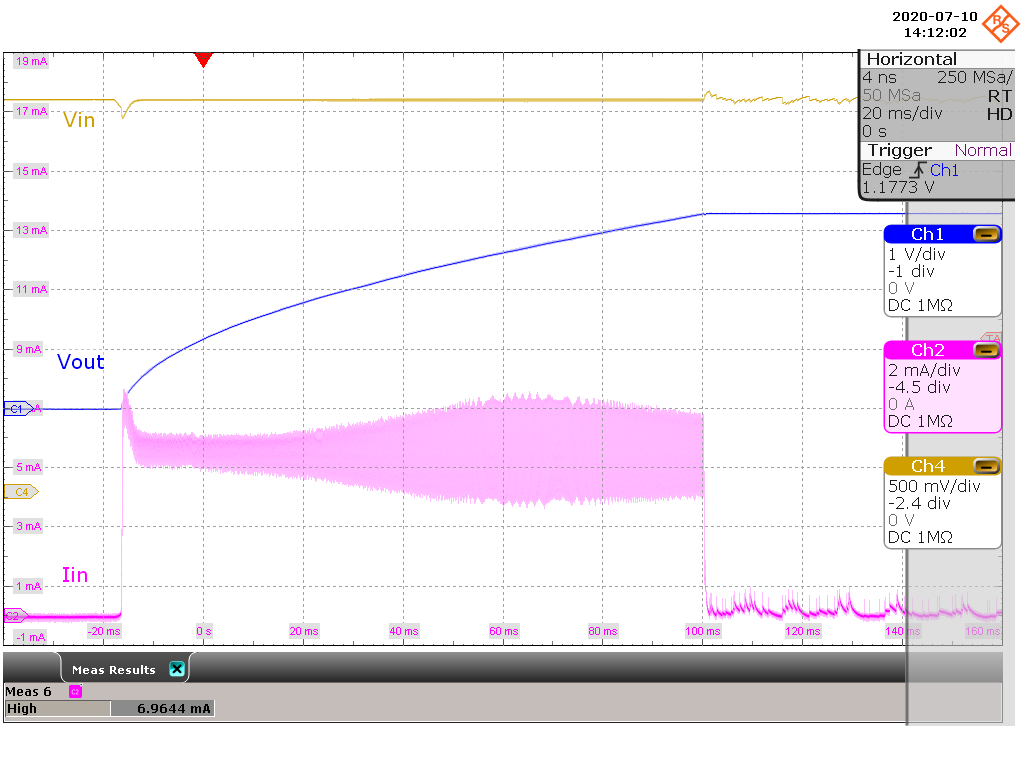VI = 3.3 V, VO = 3.3 V CI = 32 μF, CO = 300 μF
Figure 8-34 Start-up with 5-mA ICL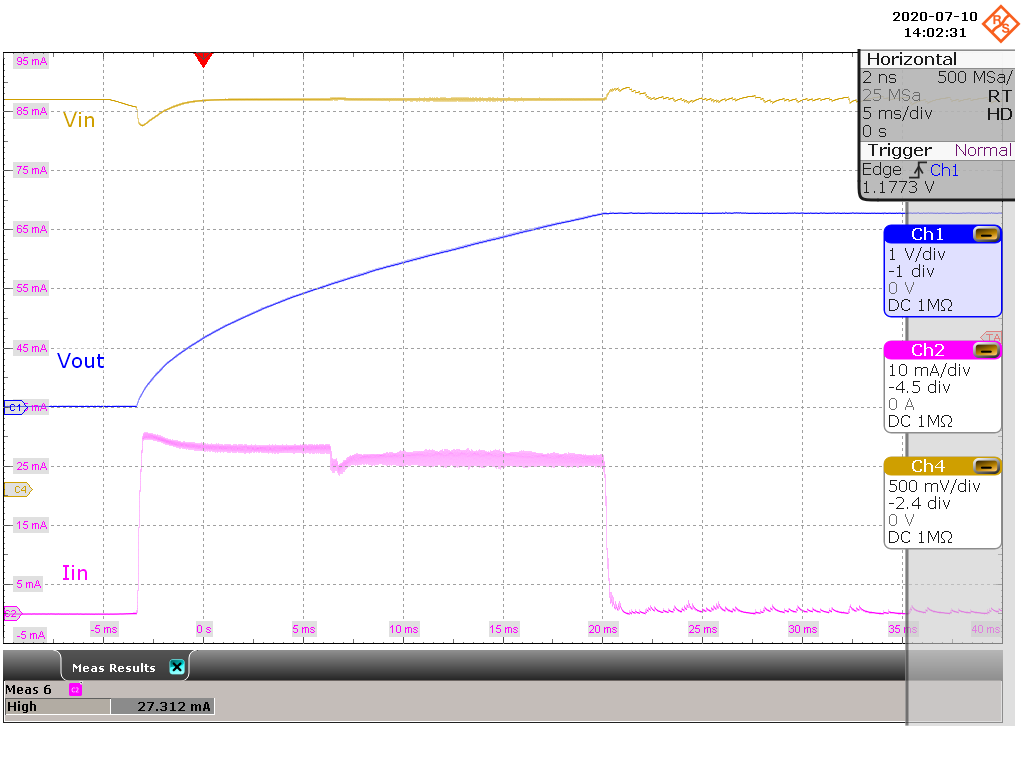VI = 3.3 V, VO = 3.3 V CI = 32 μF, CO = 300 μF
Figure 8-36 Start-up with 25-mA ICL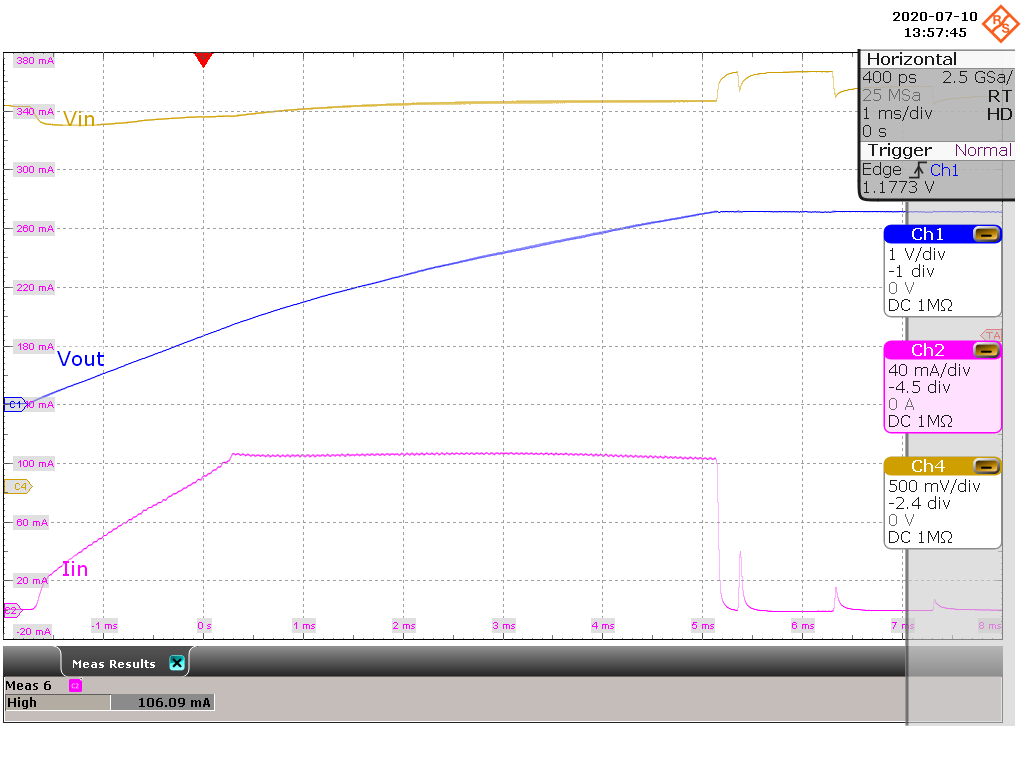VI = 3.3 V, VO = 3.3 V CI = 32 μF, CO = 300 μF
Figure 8-38 Start-up with 100-mA ICL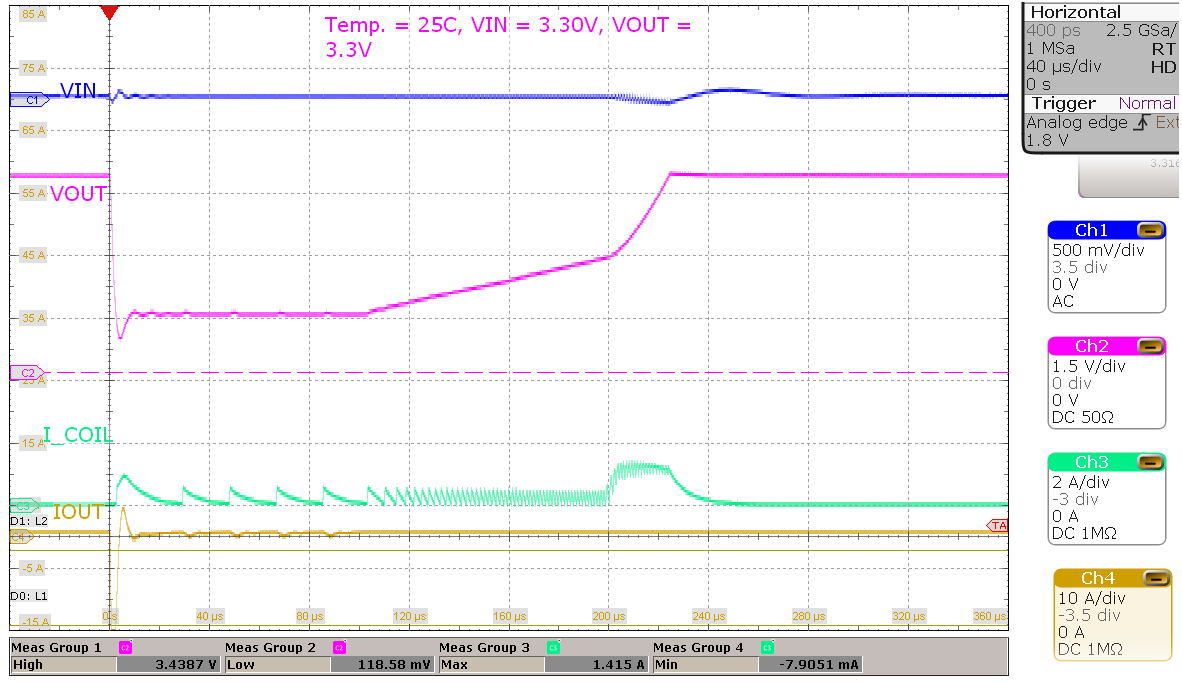VI = 3.3 V, VO = 3.3 V TA = 25°C
Figure 8-40 Short Circuit Behavior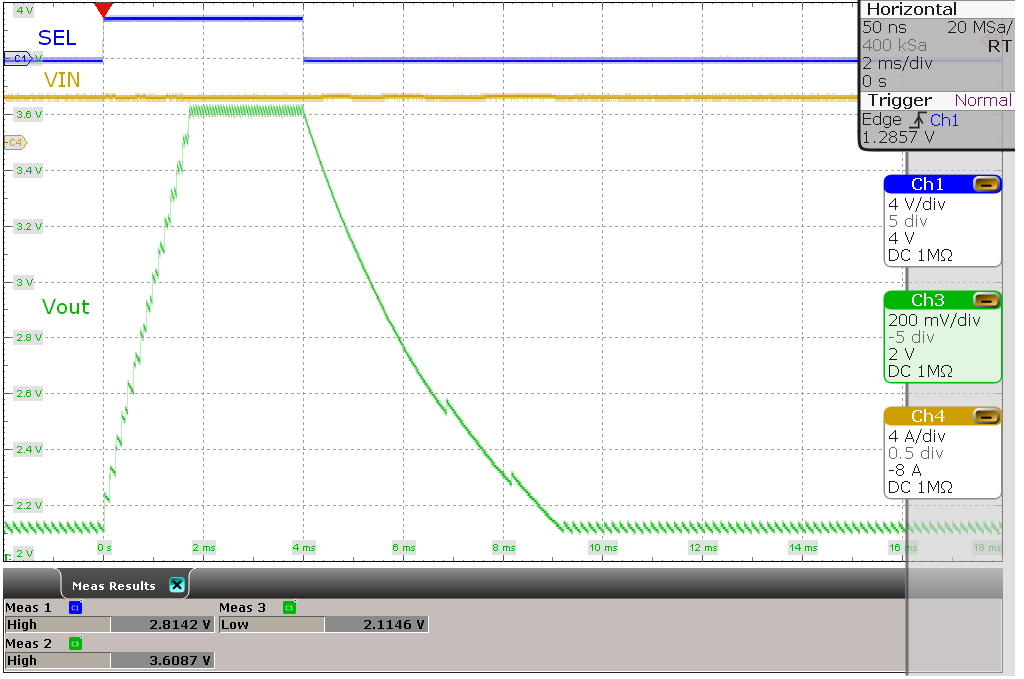VI = 3.3 V, VO(1) = 2.2 V, VO(2) = 3.6 V 1-kΩ resistive load
Figure 8-42 DVS Behavior at Light Load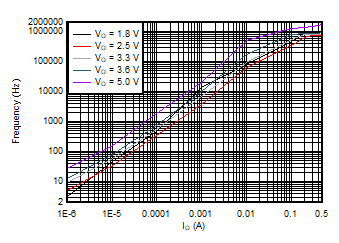VI = 3.3 V TA = 25°C
Figure 8-3 Typical Burst Switching Frequency versus Output Current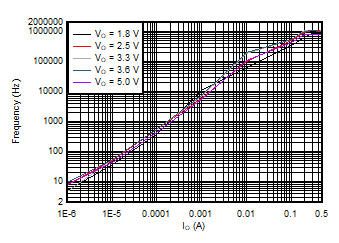VI = 5.2 V TA = 25°C
Figure 8-5 Typical Burst Switching Frequency versus Output Current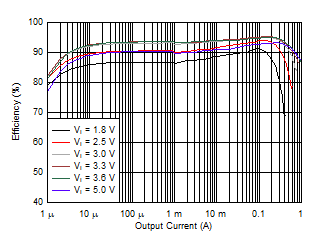VO = 3.3 V TA = 25°C
Figure 8-7 Efficiency versus Output Current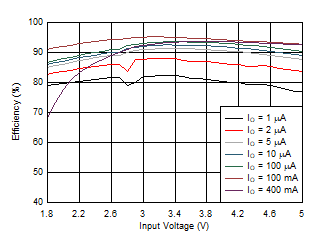VO = 3.3 V TA = 25°C
Figure 8-9 Efficiency versus Input Voltage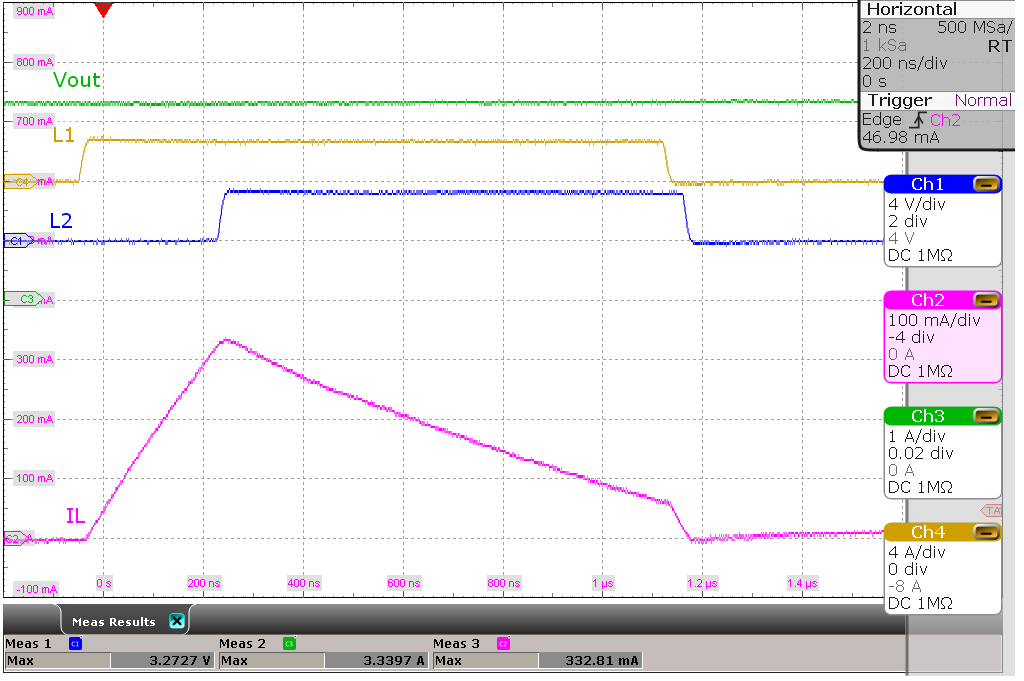VI = 2.8 V, VO = 3.3 V No load
Figure 8-11 Switching Waveforms, Boost Operation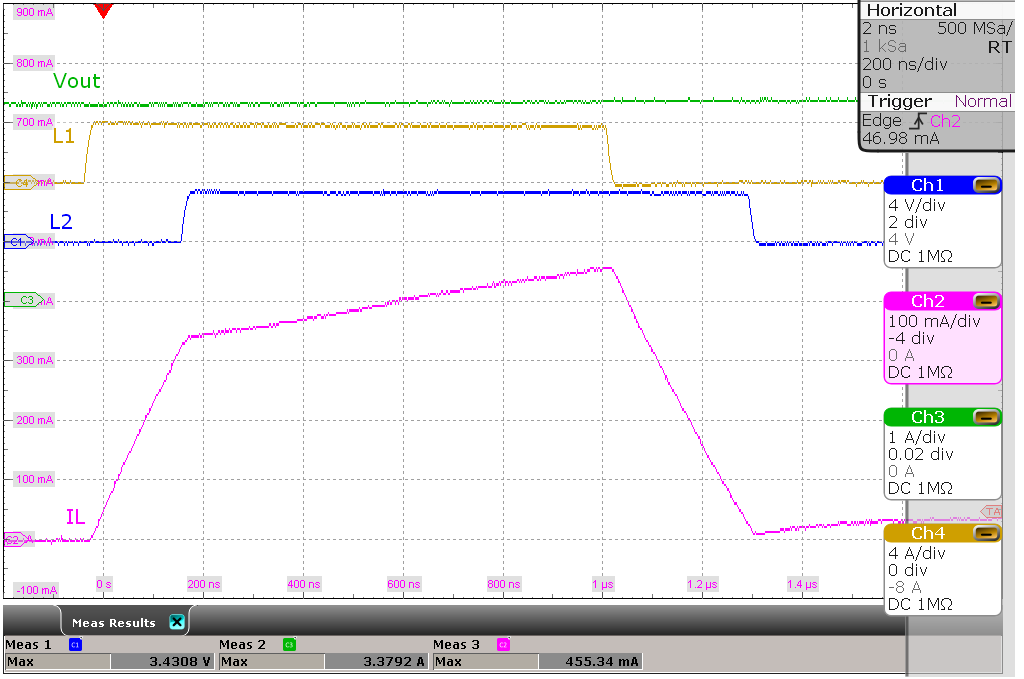VI = 4.0 V, VO = 3.3 V No load
Figure 8-13 Switching Waveforms, Buck Operation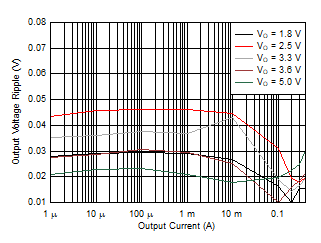VI = 3.3 V TA = 25°C
Figure 8-15 Output Voltage Ripple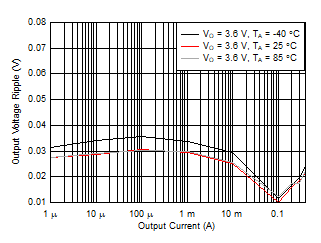VI = 3.3 V, VO = 3.6 V
Figure 8-17 Output Voltage Ripple over Temperature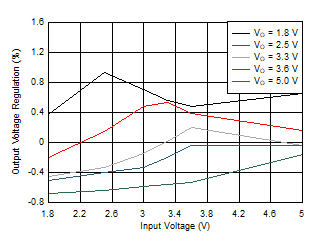VI = 1.8 V to 5.0 V Load = 1 mA, TA = 25°C
Figure 8-19 Line Regulation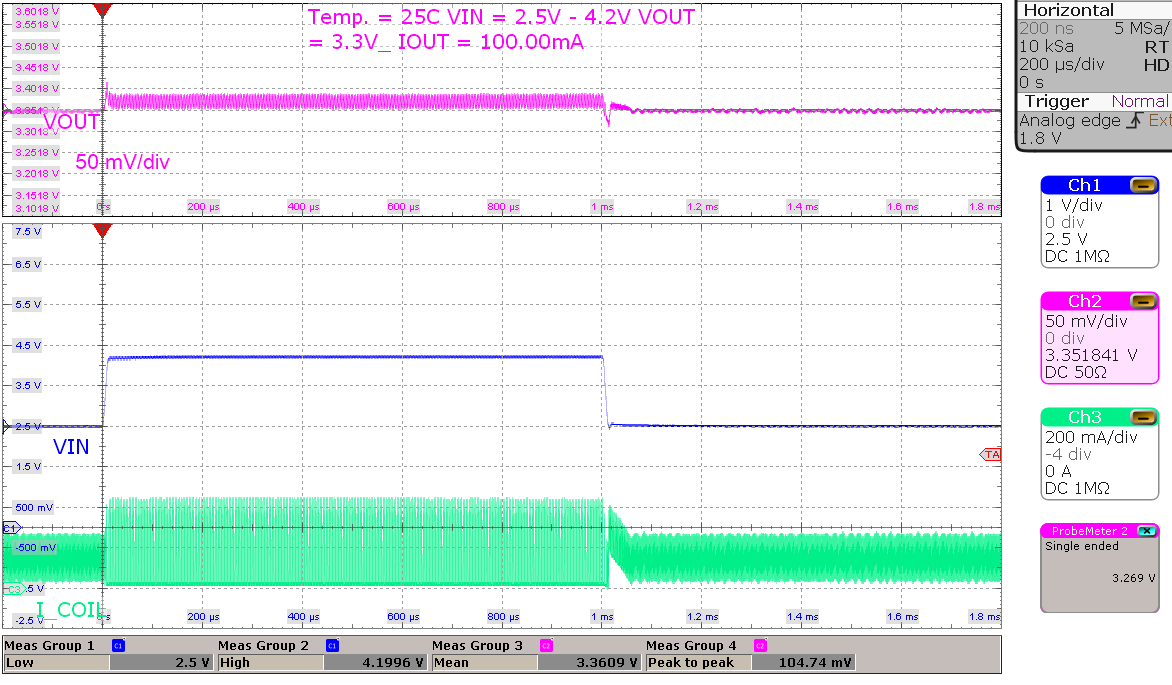VI = 2.5 V to 4.2 V, VO = 3.3 V Load = 100 mA
Figure 8-21 Line Transient, High Load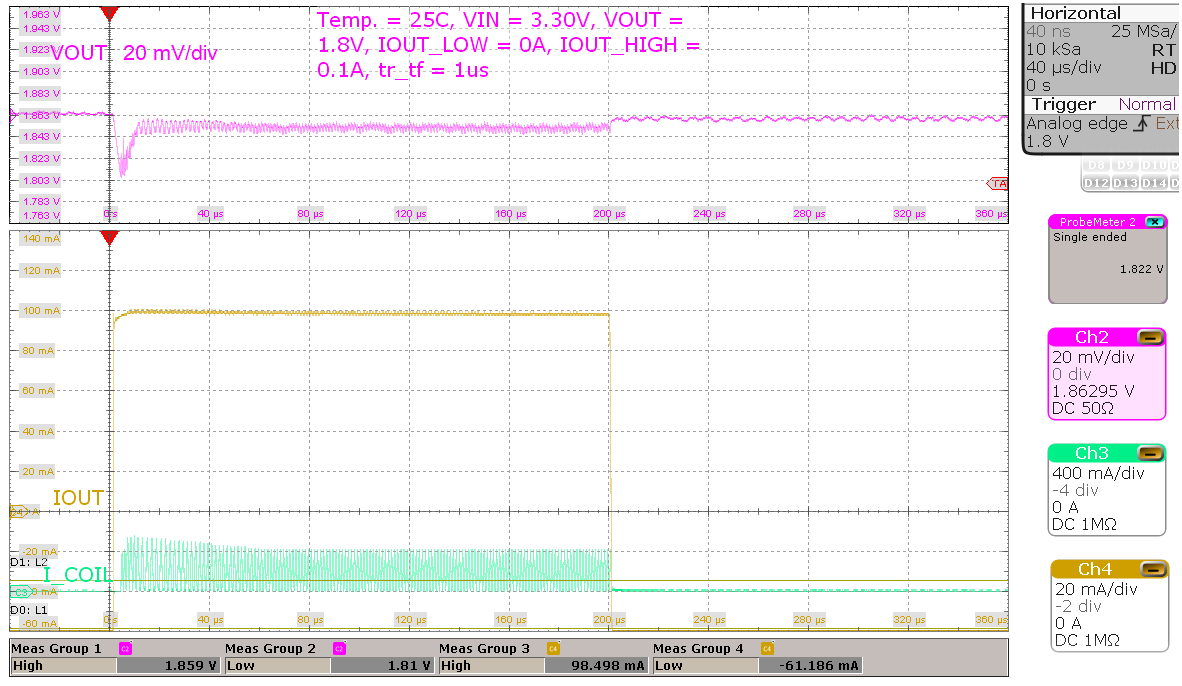VI = 3.3 V, VO = 1.8 V Load = 0 mA to 100 mA, tr/tf = 1 μs
Figure 8-23 Load Transient, 100 mA Step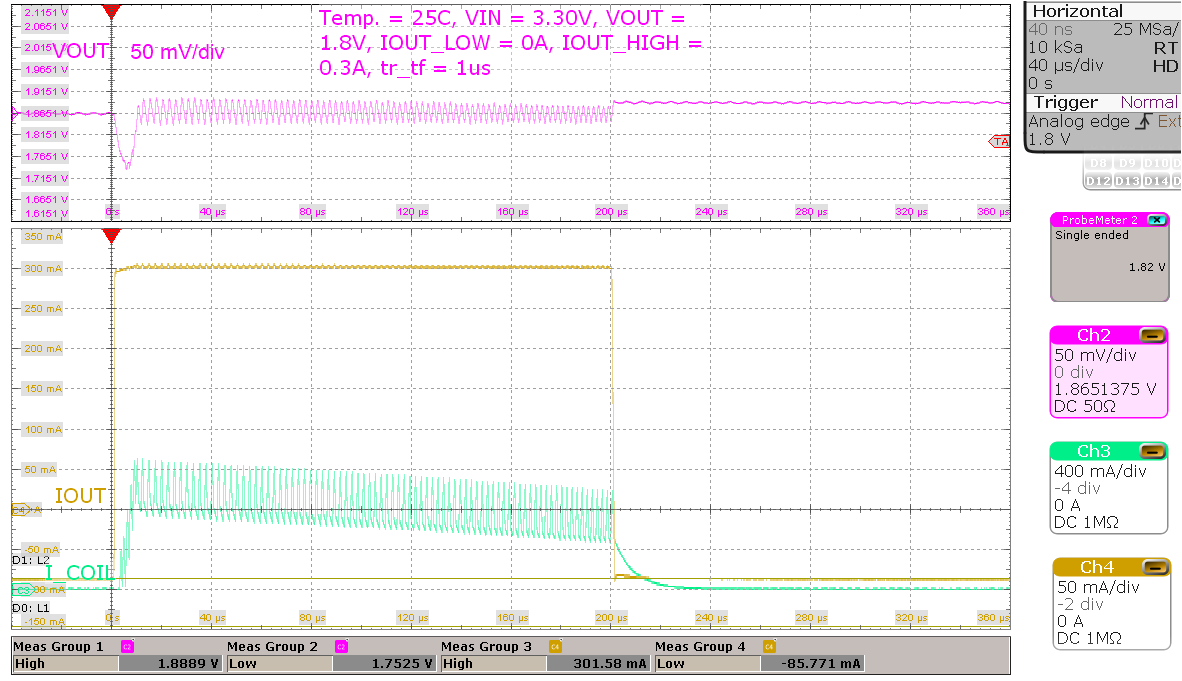VI = 3.3 V, VO = 1.8 V Load = 0 mA to 300 mA, tr/tf = 1 μs
Figure 8-25 Load Transient, 300 mA Step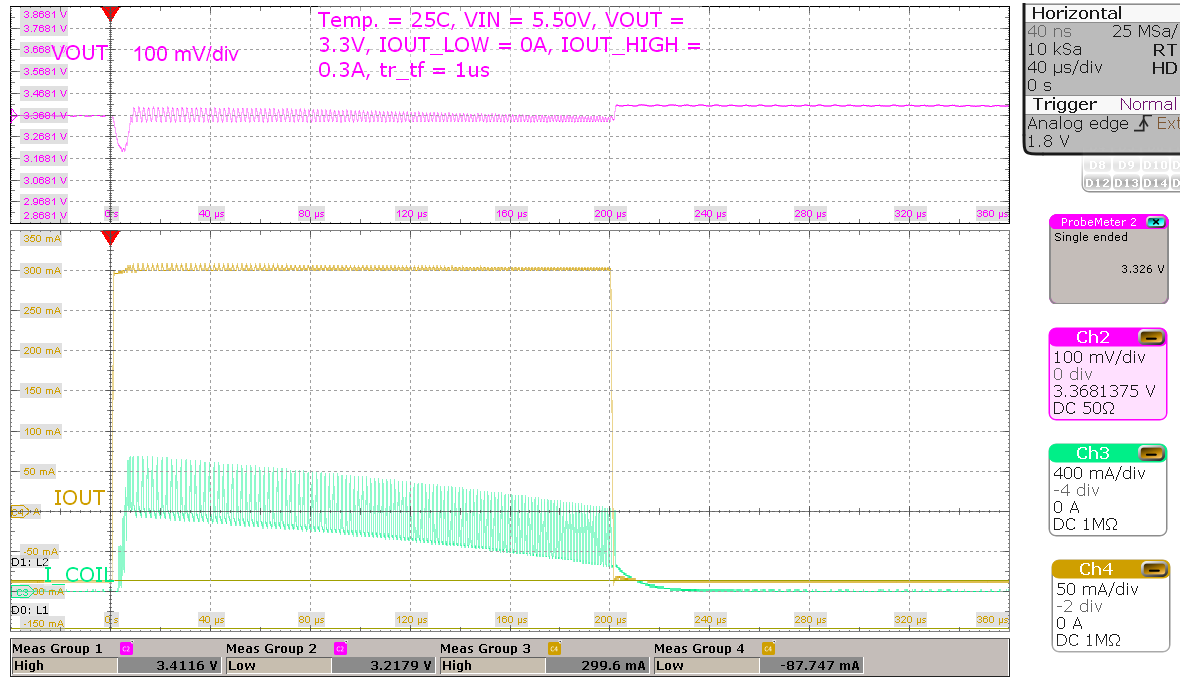VI = 5.5 V, VO = 3.3 V Load = 0 mA to 300 mA, tr/tf = 1 μs
Figure 8-27 Load Transient, 300 mA Step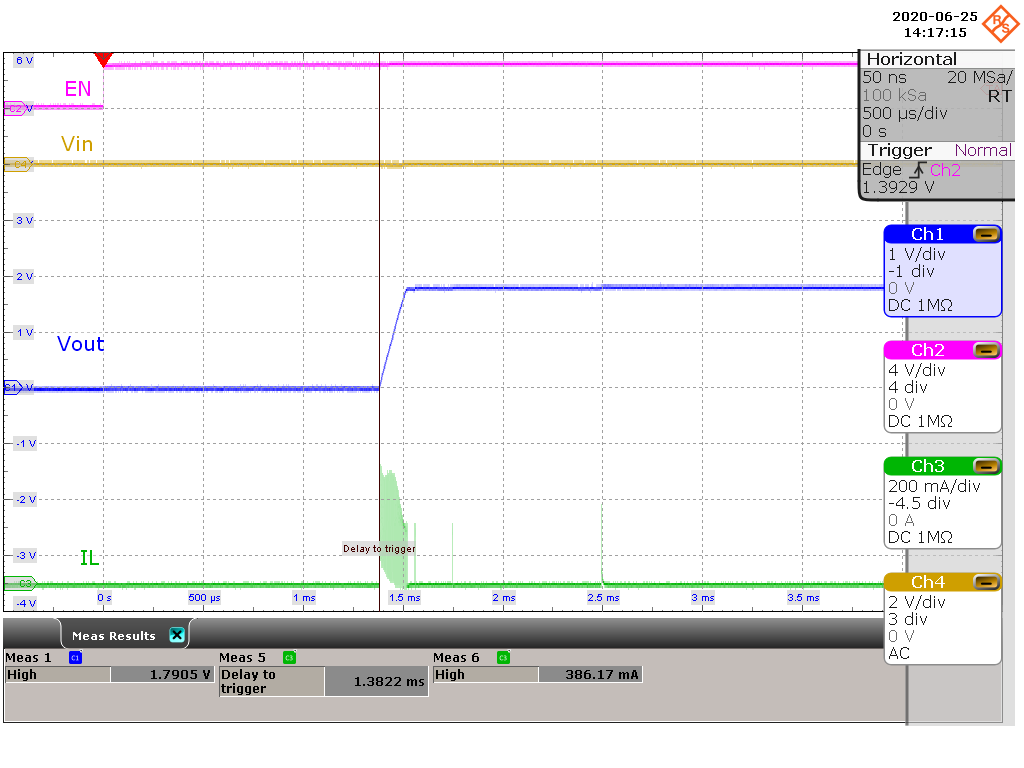VI = 1.8 V, VO = 1.8 V 10-μA resistive load
Figure 8-29 Start-up Behavior from Rising Enable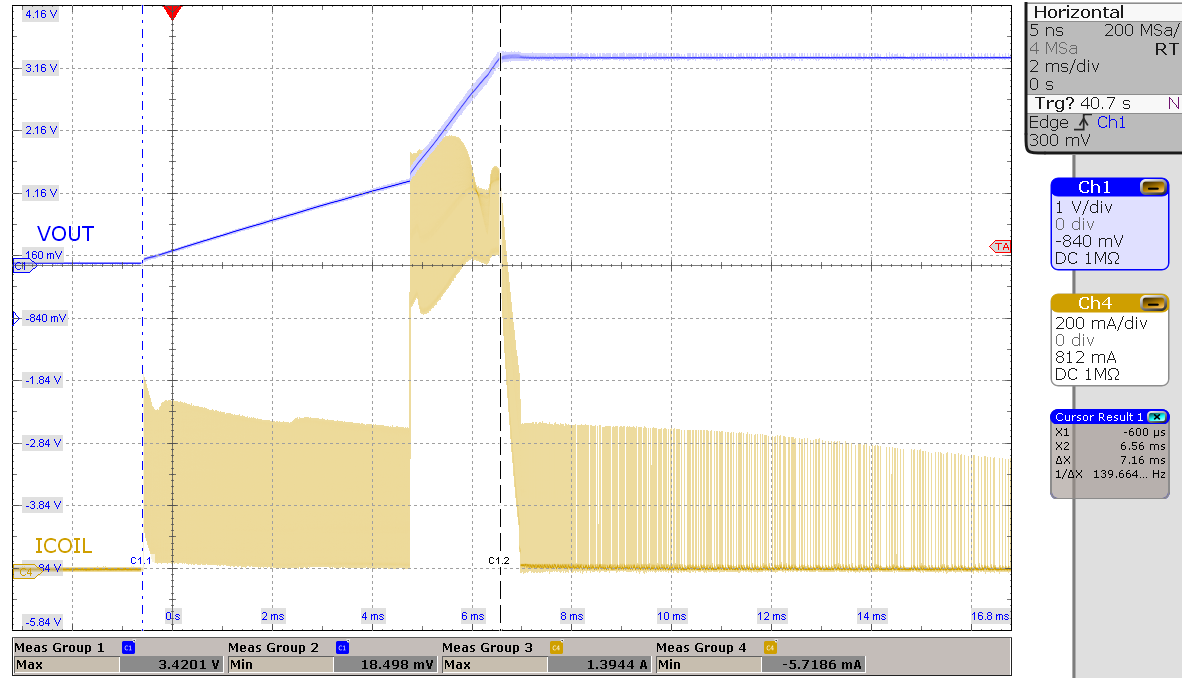VI = 3.3 V, VO = 3.3 V 1000-μF capacitive load
Figure 8-31 Start-up Behavior from Rising Enable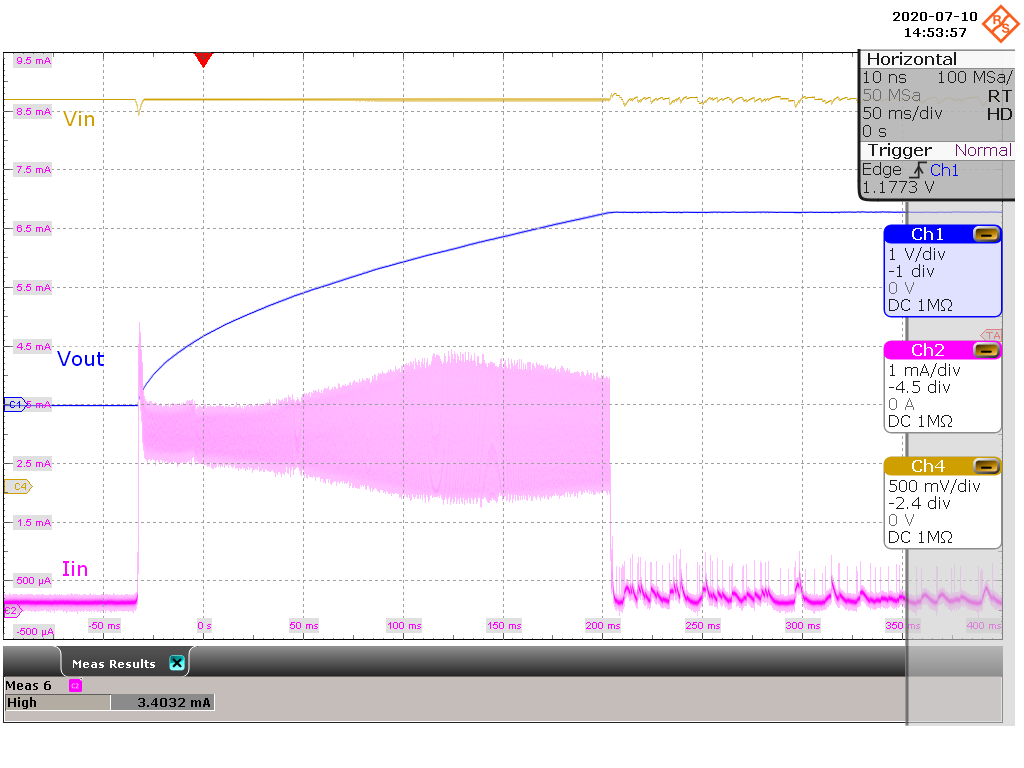VI = 3.3 V, VO = 3.3 V CI = 32 μF, CO = 300 μF
Figure 8-33 Start-up with 2.5-mA ICLVI = 3.3 V, VO = 3.3 V CI = 32 μF, CO = 300 μF
Figure 8-35 Start-up with 10-mA ICL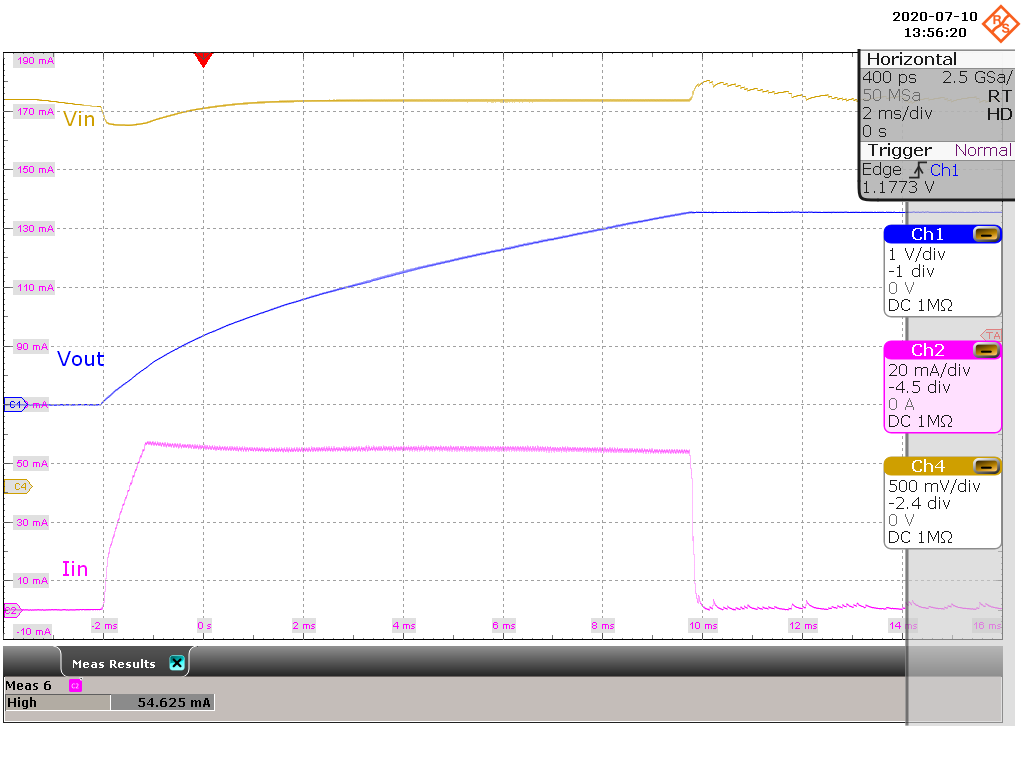VI = 3.3 V, VO = 3.3 V CI = 32 μF, CO = 300 μF
Figure 8-37 Start-up with 50-mA ICL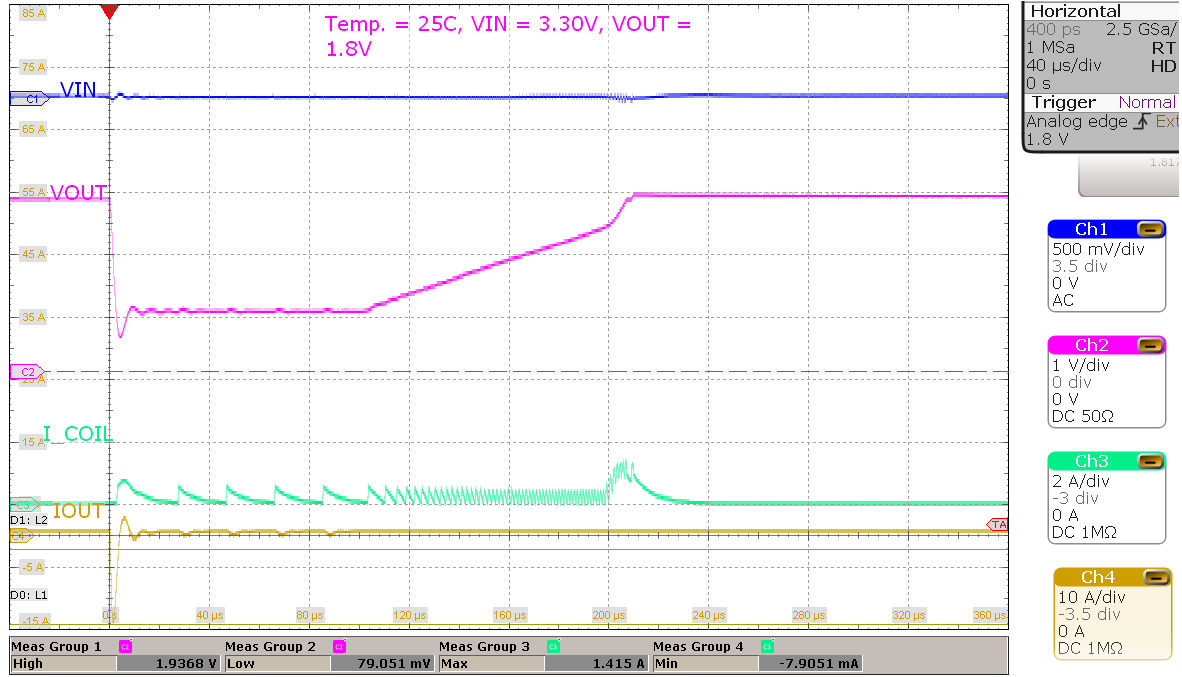VI = 3.3 V, VO = 1.8 V TA = 25°C
Figure 8-39 Short Circuit Behavior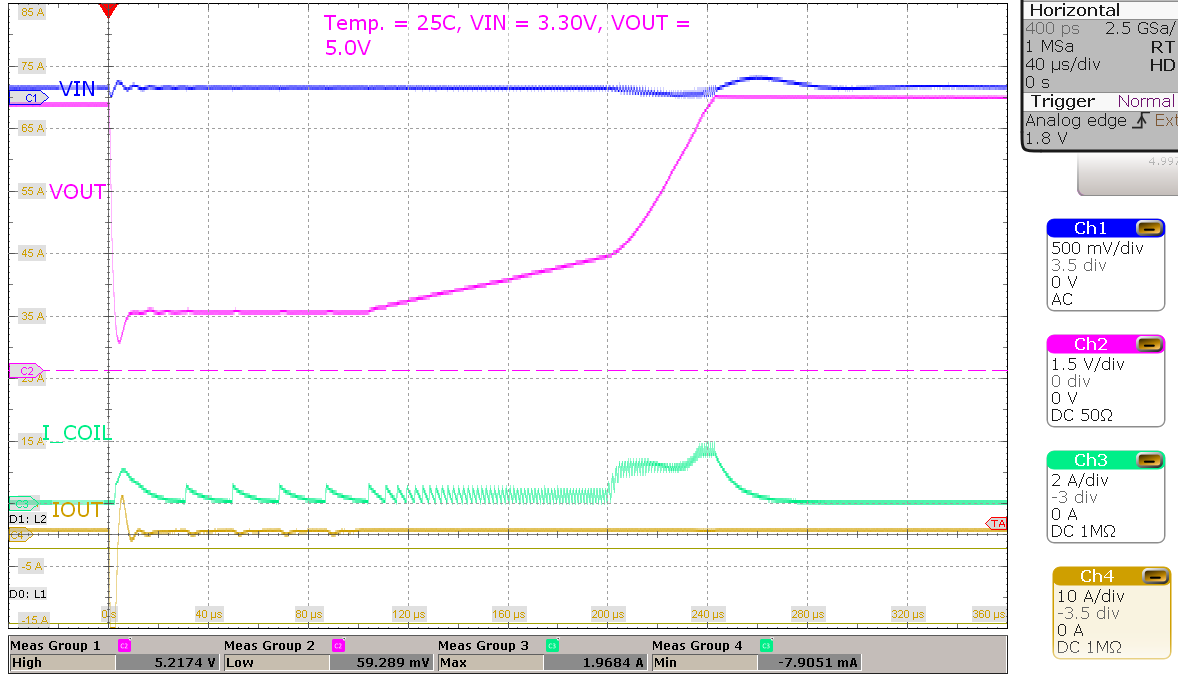VI = 3.3 V, VO = 5.0 V TA = 25°C
Figure 8-41 Short Circuit Behavior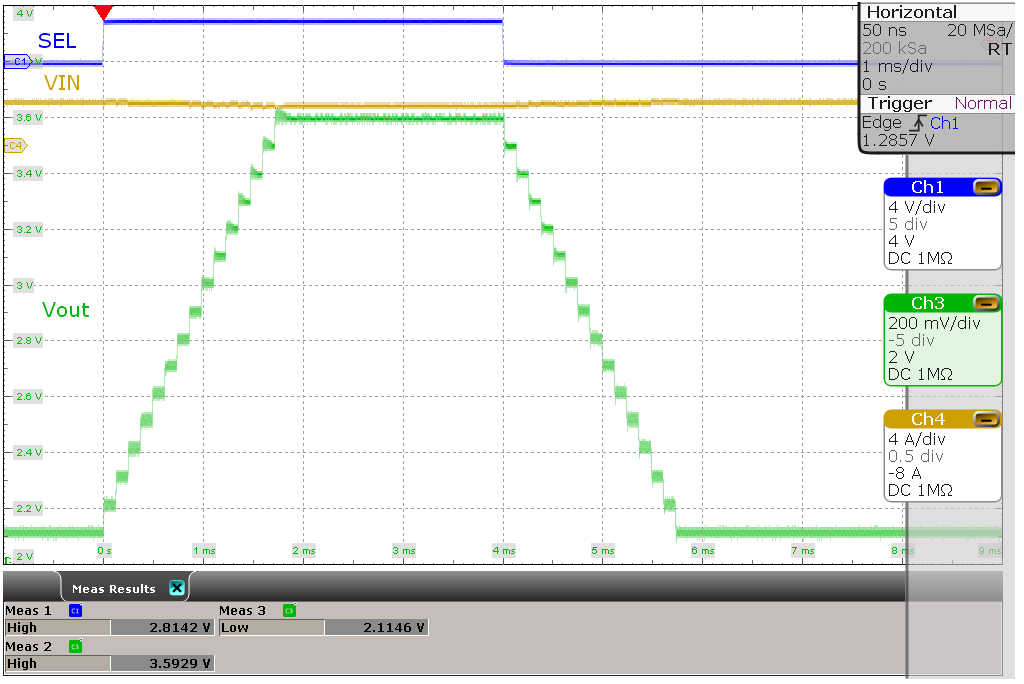VI = 3.3 V, VO(1) = 2.2 V, VO(2) = 3.6 V 30-Ω resistive load
Figure 8-43 DVS Behavior at High Load